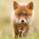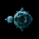1823 vues
1823
experiment with linear regression , the purpose was to catch break outs early, but it creates to much visual noise

same as version 0 but with added margin filter and signal to mark entrys
study(title="[RS]Linear Regression Bands V1", shorttitle="[RS]LRB.V1", overlay=true)
tf1 = input(0.125)
smooth = input(4)
prehh1 = nz(hh1, high)
prell1 = nz(ll1, low)

hh1 = close >= prehh1 ? high : prehh1 - sma(abs(change(high, 1)*tf1), smooth)
ll1 = close <= prell1 ? low : prell1 + sma(abs(change(low, 1)*tf1), smooth)
midline = avg(hh1, ll1)
plot(midline, style=cross, color=black, linewidth=1)
ph1 = plot(hh1, style=line, color=black, linewidth=1)
pl1 = plot(ll1, style=line, color=black, linewidth=1)

margin = input(30) * syminfo.mintick
signalcolor = high-margin > hh1 ? maroon : low+margin < ll1 ? green : gray
signal = high-margin > hh1 ? high+margin : low+margin < ll1 ? low-margin : na
plot(signal, style=circles, color=signalcolor, linewidth=4)coondawg71
:) awsome, using the 8/1 > 7/1 and 1/8 > 2/8 murreys for rejection confirmation?
RépondreRicardoSantos
sure can, there is so much going on here, it can be viewed several ways. I just took the view option off all but one band, and tweeked the periods to suit an actual time period. Since you posted with a 5 min chart, I think its smart to use periods that work with hourly, 4 hour and daily time frames, those the times that matter rather than an arbitrary period. Helps smooth the data. I added the LSMA as a baseline for the chart. I see LSMA as the magnetic force of the price action. Then I changed the band median to an actual line just so my eyes can see it more easily and how it flows across the chart. Its the thick black line. Pink line is the Murrey channel median. So we can obviously see price action bias when the bands median crosses the murrey median all while be pulled like a vortex by the LSMA. Murrey is set to 288 to signify the daily time frame. Plus i changed the input for the bands Tf1 to 0.125 for smoothness sake, the actual smoothing factor set to 12 for one hour and the margin to 48 as in four hours, most heavy traded retail time frame. Seems to complement each other well.
Répondrecoondawg71
yea i neglected to mention xD , the Tf suposed to be fractions 0.5 = 1/2 , 0.25 = 1/4, 0.125 = 1/8, 0.0625 = 1/16 ....
Répondre